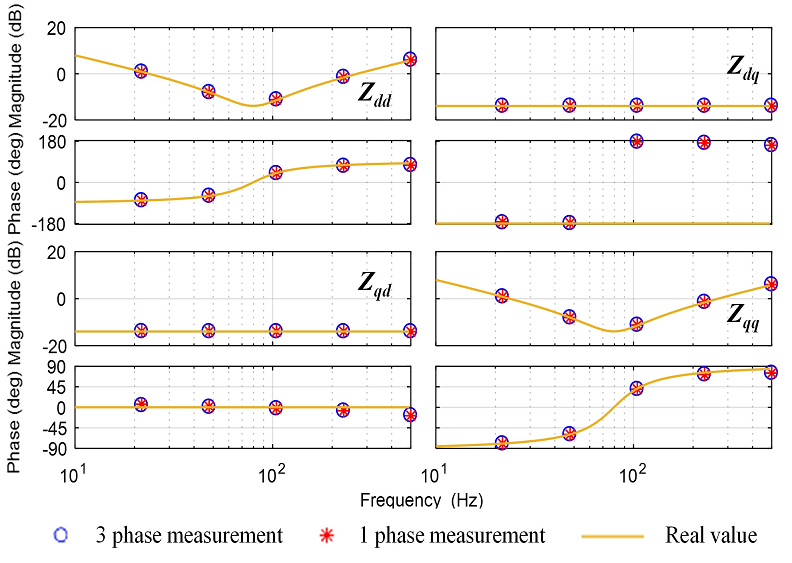LIBRARY

# A Novel DQ Impedance Measurement Method in Three-Phase Balanced SystemsFig. 1. Impedance measurement results for a passive load.
The stability of electronic power systems is essential to the safety and reliability of the system designs. Optimum stability analysis and measurement techniques are being discussed more frequently in the literature. One approach of assessing system stability is the generalized nyquist criterion (GNC), which is based on d-q frame impedances. An impedance measurement unit (IMU) can be used to acquire d-q frame impedances for a three-phase balanced system. A novel d-q frame impedance measurement method, namely the single-phase impedance measurement approach, is applied to a three-phase balanced passive circuit and a voltage source inverter (VSI) separately. In this approach, only single-phase data are needed. A comparison of measured results proves the capability of the single-phase impedance measurement method to obtain three-phase d-q frame impedances. In a passive circuit, both measurement methods only demand single-phase perturbation, as in Fig. 1. In the converter circuit, the three-phase impedance measurement method still only needs a single-phase perturbation, while the single-phase impedance measurement method requires three separate balanced, single-phase perturbations, as in Fig. 2.
The single-phase impedance measurement process has been applied to two prototype three-phase balanced systems. For passive circuits, voltage perturbations and current responses are both multiplied by the ratio of square root of 8/3 in single-phase impedance measurement compared to three-phase measurement, leading to the same impedance measurement results. For a converter system with d-q frame controls, in order to obtain accurate impedance measurement results using the single-phase impedance measurement method, three-phase balanced perturbations are required by the impedance measurement unit, regardless if the three phases are perturbed together or separately. The analysis shows that only six sensors are needed for measuring d-q impedances of three-phase balanced power systems. Compared to the traditional approach, this method will reduce the quantity of sensors. The reduction in sensors is especially beneficial for high-voltage and high-power applications where bulky and expensive current and voltage sensors are needed.Fig. 2. Single-phase measurement method on a VSI with current control under three separate phase perturbations.